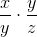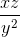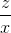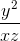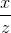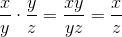Algebra 1 : Monomials

Example Questions

Example Question #141 : Monomials

Multiply the following monomial quotiemts: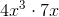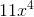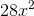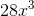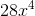Explanation:

To solve this problem, split it into two steps:

1. Multiply the coefficients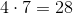2. Multiply the variables. We also need to remember the following laws of exponents rule: When multiplying variables, add the exponents.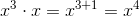Combine these to get the final answer:Example Question #142 : Monomials

Multiply the following monomial quotients: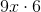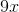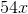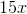Explanation:

To solve this problem, split it into two steps:

1. Multiply the coefficients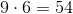2. Combine this with the lone variable to get the final answer:Example Question #143 : Monomials

Multiply the following monomial quotients: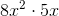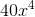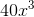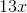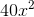Explanation:

To solve this problem, split it into two steps:

1. Multiply the coefficients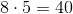2. Multiply the variables. We also need to remember the following laws of exponents rule: When multiplying variables, add the exponents.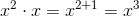Combine these to get the final answer:Example Question #144 : Monomials

Multiply the following monomial quotients: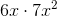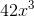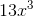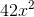Explanation:

To solve this problem, split it into two steps:

1. Multiply the coefficients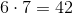2. Multiply the variables. We also need to remember the following laws of exponents rule: When multiplying variables, add the exponents.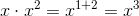Combine these to get the final answer:Example Question #145 : Monomials

Multiply the following monomial quotients: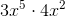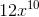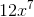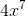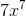Explanation:

To solve this problem, split it into two steps:

1. Multiply the coefficients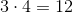2. Multiply the variables. We also need to remember the following laws of exponents rule: When multiplying variables, add the exponents.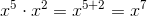Combine these to get the final answer:Example Question #34 : How To Multiply Monomial Quotients

Multiply the following monomial quotients: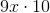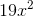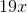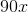Explanation:

To solve this problem, split it into two steps:

1. Multiply the coefficients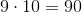2. Combine this with the lone variable e to get the final answer:Example Question #147 : Monomials

Multiply the following monomial quotients: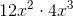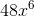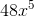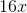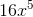Explanation:

To solve this problem, split it into two steps:

1. Multiply the coefficients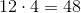2. Multiply the variables. We also need to remember the following laws of exponents rule: When multiplying variables, add the exponents.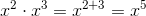Combine these to get the final answer:Example Question #571 : Variables

Multiply the following monomial quotients: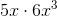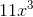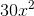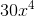Explanation:

To solve this problem, split it into two steps:

1. Multiply the coefficients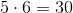2. Multiply the variables. We also need to remember the following laws of exponents rule: When multiplying variables, add the exponents.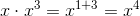Combine these to get the final answer:Example Question #572 : Variables

Multiply the following monomial quotients: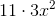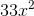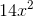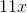Explanation:

To solve this problem, split it into two steps:

1. Multiply the coefficients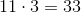2. Combine this with the lone variable to get the final answer:Example Question #573 : Variables

Simplify the following: